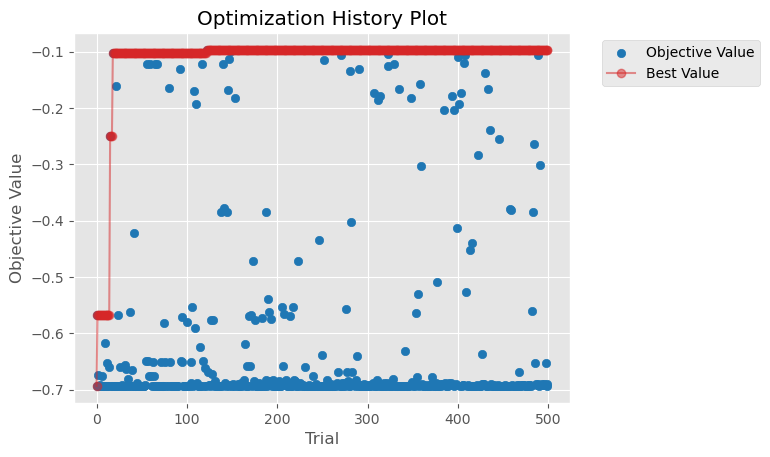# Distributed Hyperparameter Optimization with Dask and Optuna#

Optuna is a very powerful open source framework that helps automate hyperparameter search, and it integrates with Dask allowing you to run optimization trials in parallel on a cluster.

This example walks through a workload which uses Dask and Optuna, to optimize an XGBoost classification model in parallel across a Dask cluster.

You can `download this jupyter notebook` and watch the video below to follow along:

## Before you start#

You’ll first need install the necessary packages. For the purposes of this example, we’ll do this in a new virtual environment, but you could also install them in whatever environment you’re already using for your project.

```\$ conda create -n dask-optuna-example -c conda-forge python=3.10 optuna dask coiled xgboost sklearn matplotlib
```

You also could use `pip`, or any other package manager you prefer; `conda` isn’t required.

When you create a cluster, Coiled will automatically replicate your local `dask-optuna-example` environment in your cluster.

## Get a Coiled Cluster#

To start we need to spin up a Dask cluster

```import coiled

cluster = coiled.Cluster(
n_workers=20,
)
```

```from dask.distributed import Client

client = Client(cluster)
client
```

## Optuna Study#

We create a simple in-memory Optuna study and we ship it off to Dask, which will handle the coordination of the results.

The Dask integration with Optuna leverages Optuna’s existing distributed optimization capabilities to run optimization trials in parallel on a Dask cluster. It does this by providing a Dask-compatible storage `optuna.integration.DaskStorage` that can be used directly by Optuna.

Note

Usually we see people setting up a database at this step to coordinate results. However, in this case there is no need of a database, Dask can happily coordinate this (unless you already have a database handy).

```import optuna

backend_storage = optuna.storages.InMemoryStorage()

study = optuna.create_study(
direction="maximize",
sampler=optuna.samplers.RandomSampler(),
)
```

## Objective function#

In this example we use Optuna to optimize several hyperparameters for an XGBoost classifier trained on the breast cancer dataset.

There is no Dask-specific code here. This is exactly the same code you would write if you were to run Optuna on your local machine.

```import xgboost as xgb
from sklearn.model_selection import KFold, cross_val_score

def objective(trial):

params = {
"n_estimators": 10,
"verbosity": 0,
# L2 regularization weight.
"lambda": trial.suggest_float("lambda", 1e-8, 100.0, log=True),
# L1 regularization weight.
"alpha": trial.suggest_float("alpha", 1e-8, 100.0, log=True),
# sampling according to each tree.
"colsample_bytree": trial.suggest_float("colsample_bytree", 0.2, 1.0),
"max_depth": trial.suggest_int("max_depth", 2, 10, step=1),
# minimum child weight, larger the term more conservative the tree.
"min_child_weight": trial.suggest_float(
"min_child_weight", 1e-8, 100, log=True
),
"learning_rate": trial.suggest_float("learning_rate", 1e-8, 1.0, log=True),
# defines how selective algorithm is.
"gamma": trial.suggest_float("gamma", 1e-8, 1.0, log=True),
"grow_policy": "depthwise",
"eval_metric": "logloss",
}

clf = xgb.XGBClassifier(**params)
fold = KFold(n_splits=5, shuffle=True, random_state=0)
score = cross_val_score(clf, X, y, cv=fold, scoring="neg_log_loss")

return score.mean()
```

## Run optimization trials in parallel#

All of the actual coordination happens within Optuna. It’s Dask’s job just to provide a lot of firepower, which we do by submitting the optimize method many times to the cluster.

```%%time

futures = [
client.submit(study.optimize, objective, n_trials=1, pure=False) for _ in range(500)
]

_ = wait(futures)
```
```CPU times: user 1.58 s, sys: 190 ms, total: 1.77 s
Wall time: 13.5 s
```

## Results#

```study.best_params
```
```{'lambda': 1.1621290394322247e-05,
'alpha': 9.83092540863607e-05,
'colsample_bytree': 0.21935162277801334,
'max_depth': 6,
'min_child_weight': 0.8163016764545912,
'learning_rate': 0.5136962894115045,
'gamma': 2.7556478610454198e-05}
```
```study.best_value
```
```-0.09708305744830134
```
```from optuna.visualization.matplotlib import (
plot_optimization_history,
plot_param_importances,
)

plot_optimization_history(study);
```
```/var/folders/1y/ydztfpnd11b6qmvbb8_x56jh0000gn/T/ipykernel_56539/1488679482.py:6: ExperimentalWarning: plot_optimization_history is experimental (supported from v2.2.0). The interface can change in the future.
plot_optimization_history(study);
```## Clean up#

```cluster.close()
```